This is “Solve Polynomial Equations by Factoring”, section 4.4 from the book Advanced Algebra (v. 1.0). For details on it (including licensing), click here.

Has this book helped you? Consider passing it on:
Creative Commons supports free culture from music to education. Their licenses helped make this book available to you.
DonorsChoose.org helps people like you help teachers fund their classroom projects, from art supplies to books to calculators.

## 4.4 Solve Polynomial Equations by Factoring

### Learning Objectives

1. Review general strategies for factoring.
2. Solve polynomial equations by factoring.
3. Find roots of a polynomial function.
4. Find polynomial equations given the solutions.

## Reviewing General Factoring Strategies

We have learned various techniques for factoring polynomials with up to four terms. The challenge is to identify the type of polynomial and then decide which method to apply. The following outlines a general guideline for factoring polynomials:

1. Check for common factors. If the terms have common factors, then factor out the greatest common factor (GCF).
2. Determine the number of terms in the polynomial.

1. Factor four-term polynomials by grouping.
2. Factor trinomials (3 terms) using “trial and error” or the AC method.
3. Factor binomials (2 terms) using the following special products:

$Difference of squares:a2−b2=(a+b)(a−b)Sum of squares:a2+b2 no general formulaDifference of cubes:a3−b3=(a−b)(a2+ab+b2)Sum of cubes:a3+b3=(a+b)(a2−ab+b2)$
3. Look for factors that can be factored further.
4. Check by multiplying.

Note: If a binomial is both a difference of squares and a difference cubes, then first factor it as difference of squares. This will result in a more complete factorization. In addition, not all polynomials with integer coefficients factor. When this is the case, we say that the polynomial is prime.

If an expression has a GCF, then factor this out first. Doing so is often overlooked and typically results in factors that are easier to work with. Furthermore, look for the resulting factors to factor further; many factoring problems require more than one step. A polynomial is completely factored when none of the factors can be factored further.

### Example 1

Factor: $54x4−36x3−24x2+16x.$

Solution:

This four-term polynomial has a GCF of $2x.$ Factor this out first.

$54x4−36x3−24x2+16x=2x(27x3−18x2−12x+8)$

Now factor the resulting four-term polynomial by grouping and look for resulting factors to factor further.Answer: $2x(3x−2)2(3x+2).$ The check is left to the reader.

### Example 2

Factor: $x4−3x2−4.$

Solution:

This trinomial does not have a GCF.

$x4−3x2−4=(x2 )(x2 )=(x2+1)(x2−4) Difference of squares=(x2+1)(x+2)(x−2)$

The factor $(x2+1)$ is prime and the trinomial is completely factored.

Answer: $(x2+1)(x+2)(x−2)$

### Example 3

Factor: $x6+6x3−16.$

Solution:

Begin by factoring $x6=x3⋅x3$ and look for the factors of 16 that add to 6.

$x6+6x3−16=(x3 )(x3 )=(x3−2)(x3+8) sum of cubes =(x3−2)(x+2)(x2−2x+4)$

The factor $(x3−2)$ cannot be factored any further using integers and the factorization is complete.

Answer: $(x3−2)(x+2)(x2+2x+4)$

Try this! Factor: $9x4+17x2−2$

Answer: $(3x+1)(3x−1)(x2+2)$

## Solving Polynomial Equations by Factoring

In this section, we will review a technique that can be used to solve certain polynomial equations. We begin with the zero-product propertyA product is equal to zero if and only if at least one of the factors is zero.:

$a⋅b=0 if and only if a=0 or b=0$

The zero-product property is true for any number of factors that make up an equation. In other words, if any product is equal to zero, then at least one of the variable factors must be equal to zero. If an expression is equal to zero and can be factored into linear factors, then we will be able to set each factor equal to zero and solve for each equation.

### Example 4

Solve: $2x(x−4)(5x+3)=0.$

Solution:

Set each variable factor equal to zero and solve.

$2x=0 or x−4=0 or 5x+3=02x2=02x=45x5=−35x=0x=−35$

To check that these are solutions we can substitute back into the original equation to see if we obtain a true statement. Note that each solution produces a zero factor. This is left to the reader.

Answer: The solutions are 0, 4, and $−35.$

Of course, most equations will not be given in factored form.

### Example 5

Solve: $4x3−x2−100x+25=0.$

Solution:

Begin by factoring the left side completely.

$4x3−x2−100x+25=0Factor by grouping.x2(4x−1)−25(4x−1)=0(4x−1)(x2−25)=0Factor as a difference of squares.(4x−1)(x+5)(x−5)=0$

Set each factor equal to zero and solve.

$4x−1=0orx+5=0orx−5=04x=1x=−5x=5x=14$

Answer: The solutions are $14$, −5, and 5.

Using the zero-product property after factoring an equation that is equal to zero is the key to this technique. However, the equation may not be given equal to zero, and so there may be some preliminary steps before factoring. The steps required to solve by factoringThe process of solving an equation that is equal to zero by factoring it and then setting each variable factor equal to zero. are outlined in the following example.

### Example 6

Solve: $15x2+3x−8=5x−7.$

Solution:

Step 1: Express the equation in standard form, equal to zero. In this example, subtract $5x$ from and add 7 to both sides.

$15x2+3x−8=5x−715x2−2x−1=0$

Step 2: Factor the expression.

$(3x−1)(5x+1)=0$

Step 3: Apply the zero-product property and set each variable factor equal to zero.

$3x−1=0 or 5x+1=0$

Step 4: Solve the resulting linear equations.

$3x−1=0or5x+1=03x=15x=−1x=13x=−15$

Answer: The solutions are $13$ and $−15.$ The check is optional.

### Example 7

Solve: $(3x+2)(x+1)=4.$

Solution:

This quadratic equation appears to be factored; hence it might be tempting to set each factor equal to 4. However, this would lead to incorrect results. We must rewrite the equation equal to zero, so that we can apply the zero-product property.

$(3x+2)(x+1)=43x2+3x+2x+2=43x2+5x+2=43x2+5x−2=0$

Once it is in standard form, we can factor and then set each factor equal to zero.

$(3x−1)(x+2)=03x−1=0 or x+2=0 3x=1 x=−2 x=13$

Answer: The solutions are $13$ and −2.

## Finding Roots of Functions

Recall that any polynomial with one variable is a function and can be written in the form,

$f(x)=anxn+an−1xn−1+⋯+a1x+a0$

A rootA value in the domain of a function that results in zero. of a function is a value in the domain that results in zero. In other words, the roots occur when the function is equal to zero, $f(x)=0.$

### Example 8

Find the roots: $f(x)=(x+2)2−4.$

Solution:

To find roots we set the function equal to zero and solve.

$f(x)=0(x+2)2−4=0x2+4x+4−4=0x2+4x=0x(x+4)=0$

Next, set each factor equal to zero and solve.

$x=0 or x+4=0x=−4$

We can show that these x-values are roots by evaluating.

$f(0)=(0+2)2−4 f(−4)=(−4+2)2−4=4−4 =(−2)2−4=0 ✓=4−4=0 ✓$

Answer: The roots are 0 and −4.

If we graph the function in the previous example we will see that the roots correspond to the x-intercepts of the function. Here the function $f$ is a basic parabola shifted 2 units to the left and 4 units down.### Example 9

Find the roots: $f(x)=x4−5x2+4.$

Solution:

To find roots we set the function equal to zero and solve.

$f(x)=0x4−5x2+4=0(x2−1)(x2−4)=0(x+1)(x−1)(x+2)(x−2)=0$

Next, set each factor equal to zero and solve.

$x+1=0 or x−1=0 or x+2=0 or x−2=0x=−1x=1x=−2x=2$

Answer: The roots are −1, 1, −2, and 2.

Graphing the previous function is not within the scope of this course. However, the graph is provided below:Notice that the degree of the polynomial is 4 and we obtained four roots. In general, for any polynomial function with one variable of degree n, the fundamental theorem of algebraGuarantees that there will be as many (or fewer) roots to a polynomial function with one variable as its degree. guarantees n real roots or fewer. We have seen that many polynomials do not factor. This does not imply that functions involving these unfactorable polynomials do not have real roots. In fact, many polynomial functions that do not factor do have real solutions. We will learn how to find these types of roots as we continue in our study of algebra.

### Example 10

Find the roots: $f(x)=−x2+10x−25.$

Solution:

To find roots we set the function equal to zero and solve.

$f(x)=0−x2+10x−25=0−(x2−10x+25)=0−(x−5)(x−5)=0$

Next, set each variable factor equal to zero and solve.

$x−5=0orx−5=0=5x=5$

A solution that is repeated twice is called a double rootA root that is repeated twice.. In this case, there is only one solution.

The previous example shows that a function of degree 2 can have one root. From the factoring step, we see that the function can be written

$f(x)=−(x−5)2$

In this form, we can see a reflection about the x-axis and a shift to the right 5 units. The vertex is the x-intercept, illustrating the fact that there is only one root.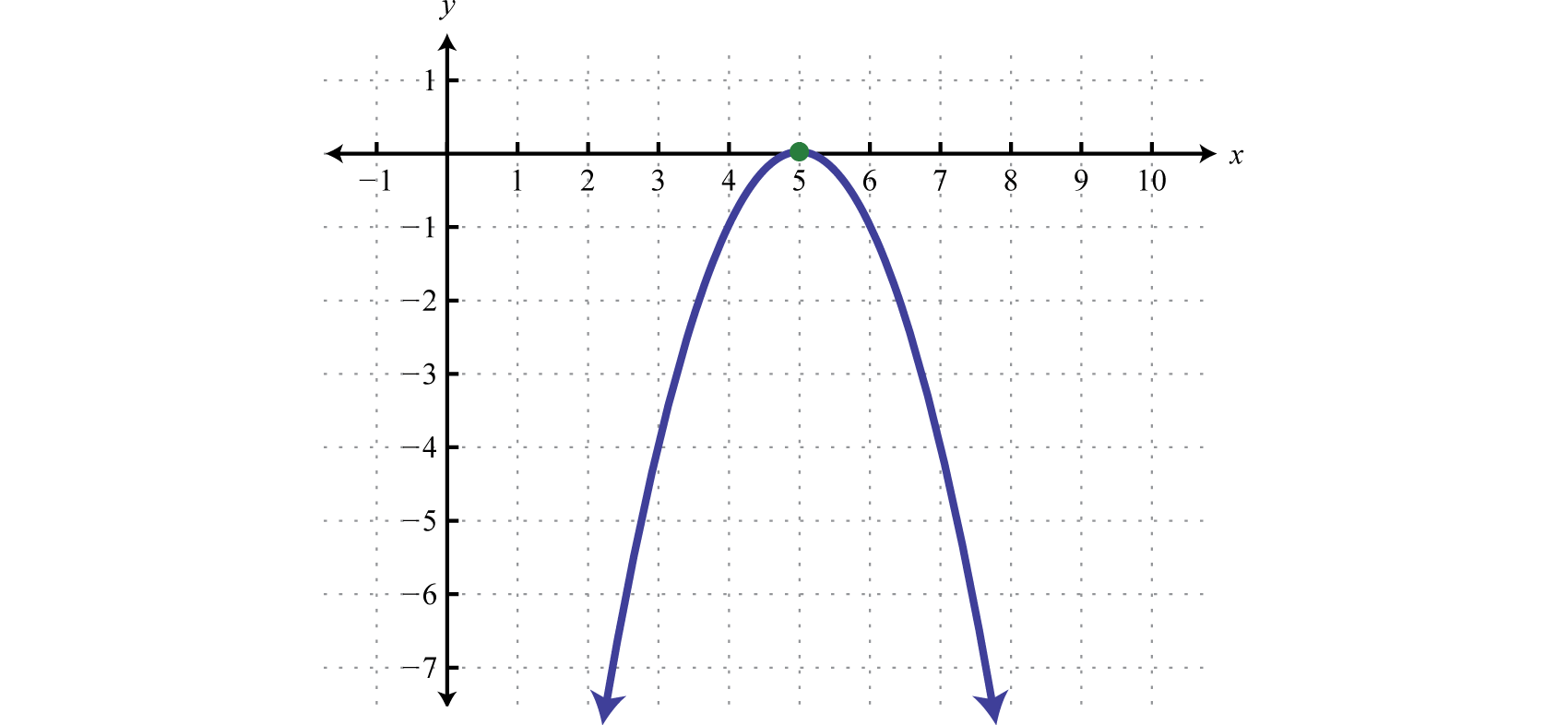Try this! Find the roots of $f(x)=x3+3x2−x−3.$

### Example 11

Assuming dry road conditions and average reaction times, the safe stopping distance in feet is given by $d(x)=120x2+x$, where x represents the speed of the car in miles per hour. Determine the safe speed of the car if you expect to stop in 40 feet.

Solution:

We are asked to find the speed x where the safe stopping distance $d(x)=40$ feet.

$d(x)=40120x2+x=40$

To solve for x, rewrite the resulting equation in standard form. In this case, we will first multiply both sides by 20 to clear the fraction.

$20(120x2+x)=20(40)x2+20x=800x2+20x−800=0$

Next factor and then set each factor equal to zero.

$x2+20x−800=0(x+40)(x−20)=0x+40=0orx−20=0x=−40x=20$

The negative answer does not make sense in the context of this problem. Consider $x=20$ miles per hour to be the only solution.

## Finding Equations with Given Solutions

We can use the zero-product property to find equations, given the solutions. To do this, the steps for solving by factoring are performed in reverse.

### Example 12

Find a quadratic equation with integer coefficients, given solutions $−32$ and $13.$

Solution:

Given the solutions, we can determine two linear factors. To avoid fractional coefficients, we first clear the fractions by multiplying both sides by the denominator.

$x=−32orx=132x=−33x=12x+3=03x−1=0$

The product of these linear factors is equal to zero when $x=−32$ or $x=13.$

$(2x+3)(3x−1)=0$

Multiply the binomials and present the equation in standard form.

$6x2−2x+9x−3=06x2+7x−3=0$

We may check our equation by substituting the given answers to see if we obtain a true statement. Also, the equation found above is not unique and so the check becomes essential when our equation looks different from someone else’s. This is left as an exercise.

Answer: $6x2+7x−3=0$

### Example 13

Find a polynomial function with real roots 1, −2, and 2.

Solution:

Given solutions to $f(x)=0$ we can find linear factors.

$x=1orx=−2orx=2x−1=0x+2=0x−2=0$

Apply the zero-product property and multiply.

$(x−1)(x+2)(x−2)=0(x−1)(x2−4)=0x3−4x−x2+4=0x3−x2−4x+4=0$

Answer: $f(x)=x3−x2−4x+4$

Try this! Find a polynomial equation with integer coefficients, given solutions $12$ and $−34.$

Answer: $8x2+2x−3=0$

### Key Takeaways

• Factoring and the zero-product property allow us to solve equations.
• To solve a polynomial equation, first write it in standard form. Once it is equal to zero, factor it and then set each variable factor equal to zero. The solutions to the resulting equations are the solutions to the original.
• Not all polynomial equations can be solved by factoring. We will learn how to solve polynomial equations that do not factor later in the course.
• A polynomial function can have at most a number of real roots equal to its degree. To find roots of a function, set it equal to zero and solve.
• To find a polynomial equation with given solutions, perform the process of solving by factoring in reverse.

### Part A: General Factoring

Factor completely.

1. $50x2−18$

2. $12x3−3x$

3. $10x3+65x2−35x$

4. $15x4+7x3−4x2$

5. $6a4b−15a3b2−9a2b3$

6. $8a3b−44a2b2+20ab3$

7. $36x4−72x3−4x2+8x$

8. $20x4+60x3−5x2−15x$

9. $3x5+2x4−12x3−8x2$

10. $10x5−4x4−90x3+36x2$

11. $x4−23x2−50$

12. $2x4−31x2−16$

13. $−2x5−6x3+8x$

14. $−36x5+69x3+27x$

15. $54x5−78x3+24x$

16. $4x6−65x4+16x2$

17. $x6−7x3−8$

18. $x6−25x3−54$

19. $3x6+4x3+1$

20. $27x6−28x3+1$

### Part B: Solving Polynomial Equations by Factoring

Solve.

1. $(6x−5)(x+7)=0$

2. $(x+9)(3x−8)=0$

3. $5x(2x−5)(3x+1)=0$

4. $4x(5x−1)(2x+3)=0$

5. $(x−1)(2x+1)(3x−5)=0$

6. $(x+6)(5x−2)(2x+9)=0$

7. $(x+4)(x−2)=16$

8. $(x+1)(x−7)=9$

9. $(6x+1)(x+1)=6$

10. $(2x−1)(x−4)=39$

11. $x2−15x+50=0$

12. $x2+10x−24=0$

13. $3x2+2x−5=0$

14. $2x2+9x+7=0$

15. $110x2−715x−16=0$

16. $14−49x2=0$

17. $6x2−5x−2=30x+4$

18. $6x2−9x+15=20x−13$

19. $5x2−23x+12=4(5x−3)$

20. $4x2+5x−5=15(3−2x)$

21. $(x+6)(x−10)=4(x−18)$

22. $(x+4)(x−6)=2(x+4)$

23. $4x3−14x2−30x=0$

24. $9x3+48x2−36x=0$

25. $13x3−34x=0$

26. $12x3−150x=0$

27. $−10x3−28x2+48x=0$

28. $−2x3+15x2+50x=0$

29. $2x3−x2−72x+36=0$

30. $4x3−32x2−9x+72=0$

31. $45x3−9x2−5x+1=0$

32. $x3−3x2−x+3=0$

33. $x4−5x2+4=0$

34. $4x4−37x2+9=0$

Find the roots of the given functions.

1. $f(x)=x2+10x−24$

2. $f(x)=x2−14x+48$

3. $f(x)=−2x2+7x+4$

4. $f(x)=−3x2+14x+5$

5. $f(x)=16x2−40x+25$

6. $f(x)=9x2−12x+4$

7. $g(x)=8x2+3x$

8. $g(x)=5x2−30x$

9. $p(x)=64x2−1$

10. $q(x)=4x2−121$

11. $f(x)=15x3−1x2−120x+14$

12. $f(x)=13x3+12x2−43x−2$

13. $g(x)=x4−13x2+36$

14. $g(x)=4x4−13x2+9$

15. $f(x)=(x+5)2−1$

16. $g(x)=−(x+5)2+9$

17. $f(x)=−(3x−5)2$

18. $g(x)=−(x+2)2+4$

Given the graph of a function, determine the real roots.

1.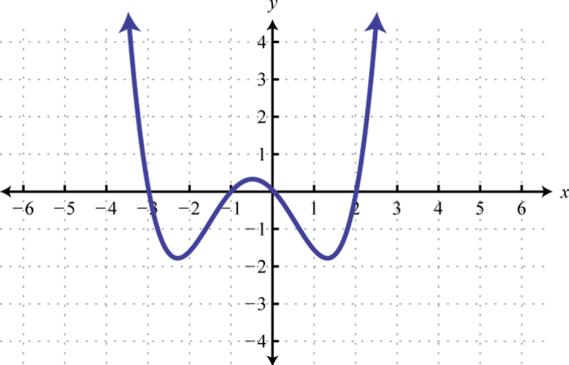2.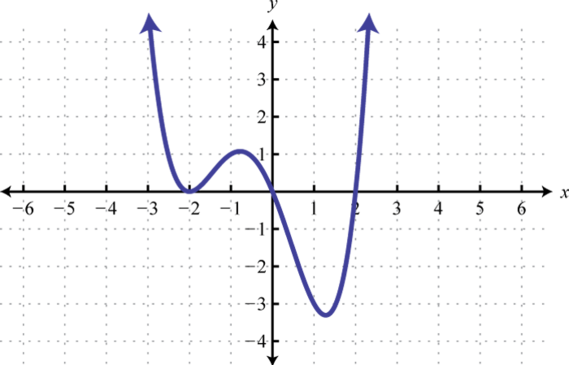3.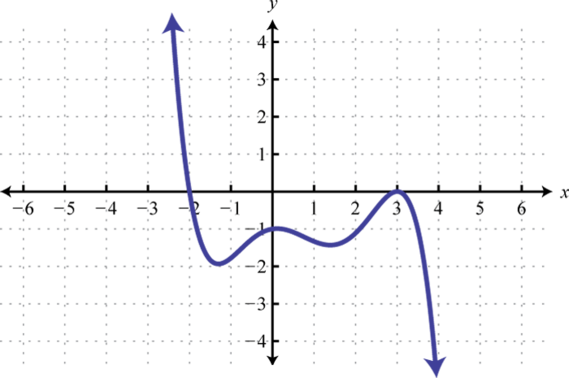4.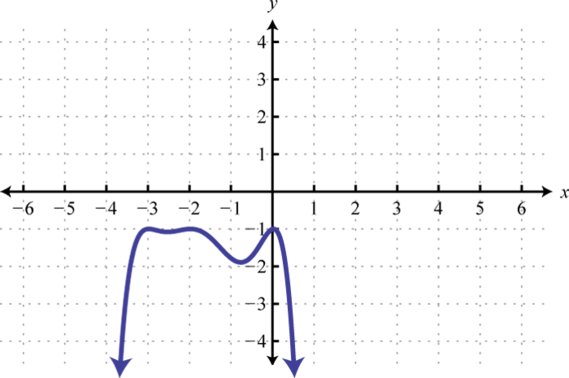5. The sides of a square measure $x−2$ units. If the area is 36 square units, then find x.

6. The sides of a right triangle have lengths that are consecutive even integers. Find the lengths of each side. (Hint: Apply the Pythagorean theorem)

7. The profit in dollars generated by producing and selling n bicycles per week is given by the formula $P(n)=−5n2+400n−6000.$ How many bicycles must be produced and sold to break even?

8. The height in feet of an object dropped from the top of a 64-foot building is given by $h(t)=−16t2+64$ where t represents the time in seconds after it is dropped. How long will it take to hit the ground?

9. A box can be made by cutting out the corners and folding up the edges of a square sheet of cardboard. A template for a cardboard box of height 2 inches is given.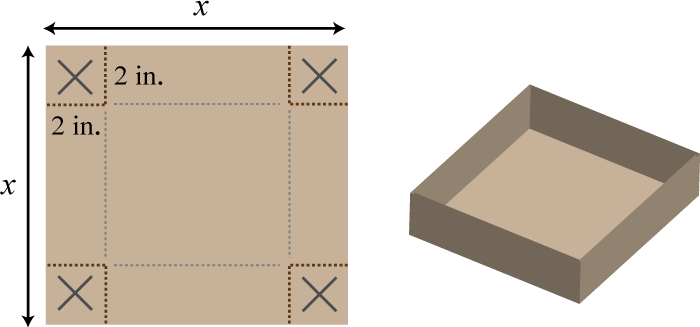What is the length of each side of the cardboard sheet if the volume of the box is to be 98 cubic inches?

10. The height of a triangle is 4 centimeters less than twice the length of its base. If the total area of the triangle is 48 square centimeters, then find the lengths of the base and height.

11. A uniform border is to be placed around an $8×10$ inch picture.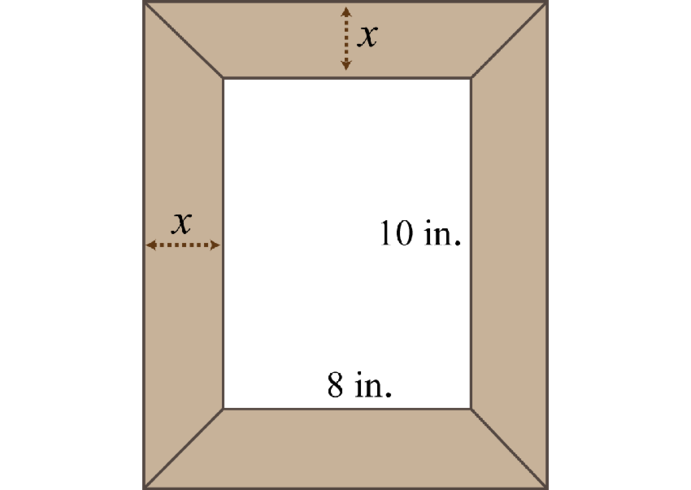If the total area including the border must be 168 square inches, then how wide should the border be?

12. The area of a picture frame including a 3-inch wide border is 120 square inches.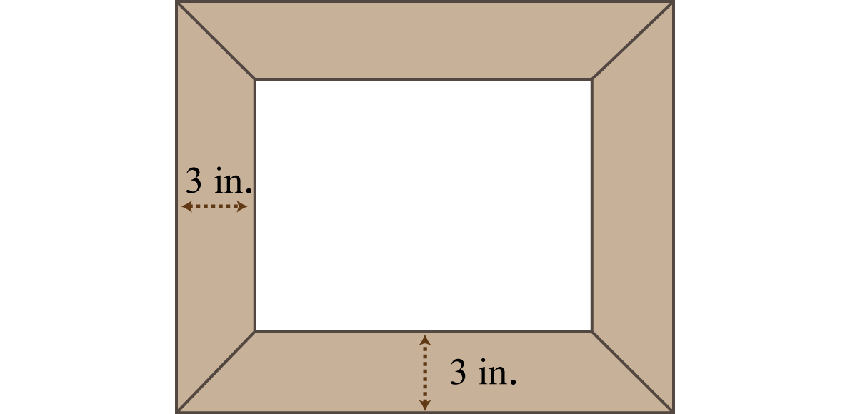If the width of the inner area is 2 inches less than its length, then find the dimensions of the inner area.

13. Assuming dry road conditions and average reaction times, the safe stopping distance in feet is given by $d(x)=120x2+x$ where x represents the speed of the car in miles per hour. Determine the safe speed of the car if you expect to stop in 75 feet.

14. A manufacturing company has determined that the daily revenue in thousands of dollars is given by the formula $R(n)=12n−0.6n2$ where n represents the number of palettes of product sold $(0≤n<20)$. Determine the number of palettes sold in a day if the revenue was 45 thousand dollars.

### Part C: Finding Equations with Given Solutions

Find a polynomial equation with the given solutions.

1. −3, 5

2. −1, 8

3. 2, $13$

4. $−34$, 5

5. 0, −4

6. 0,7

7. ±7

8. ±2

9. −3, 1, 3

10. −5, −1, 1

Find a function with the given roots.

1. $12$, $23$

2. $25$, $−13$

3. $±34$

4. $±52$

5. 5 double root

6. −3 double root

7. −1, 0, 3

8. −5, 0, 2

Recall that if $|X|=p$, then $X=−p$ or $X=p.$ Use this to solve the following absolute value equations.

1. $|x2−8|=8$

2. $|2x2−9|=9$

3. $|x2−2x−1|=2$

4. $|x2−8x+14|=2$

5. $|2x2−4x−7|=9$

6. $|x2−3x−9|=9$

### Part D: Discussion Board

1. Explain to a beginning algebra student the difference between an equation and an expression.

2. What is the difference between a root and an x-intercept? Explain.

3. Create a function with three real roots of your choosing. Graph it with a graphing utility and verify your results. Share your function on the discussion board.

4. Research and discuss the fundamental theorem of algebra.

1. $2(5x+3)(5x−3)$

2. $5x(x+7)(2x−1)$

3. $3a2b(2a+b)(a−3b)$

4. $4x(x−2)(3x+1)(3x−1)$

5. $x2(3x+2)(x+2)(x−2)$

6. $(x2+2)(x+5)(x−5)$

7. $−2x(x2+4)(x−1)(x+1)$
8. $6x(x+1)(x−1)(3x+2)(3x−2)$

9. $(x+1)(x2−x+1)(x−2)(x2+2x+4)$

10. $(3x3+1)(x+1)(x2−x+1)$

1. −7, $56$

2. 0, $52$, $−13$

3. $−12$, 1, $53$

4. −6, 4

5. $−53$, $12$

6. 5, 10

7. $−53$, 1

8. $−13$, 5

9. $−16$, 6

10. $35$, 8

11. 2, 6

12. 0, $−32$, 5

13. 0, $±32$

14. −4, 0, $65$

15. ±6, $12$

16. $±13$, $15$

17. ±1, ±2

18. 2, −12

19. $−12$, 4

20. $54$

21. $−38$, 0

22. $±18$

23. $±12$, 5

24. ±2, ±3

25. −6, −4

26. $53$

27. −3, −1, 0, 2

28. −2, 3

29. 8 units

30. 20 or 60 bicycles

31. 11 in

32. 2 inches

33. 30 miles per hour

1. $x2−2x−15=0$

2. $3x2−7x+2=0$

3. $x2+4x=0$

4. $x2−49=0$

5. $x3−x2−9x+9=0$

6. $f(x)=6x2−7x+2$

7. $f(x)=16x2−9$

8. $f(x)=x2−10x+25$

9. $f(x)=x3−2x2−3x$

10. ±4, 0

11. ±1, 3

12. −2, 1, 4